Next: Worked example 3.3: Cannon Up: Motion in 3 dimensions Previous: Worked example 3.1: Broken

## Worked example 3.2: Gallileo's experiment

Question: Legend has it that Gallileo tested out his newly developed theory of projectile motion by throwing weights from the top of the Leaning Tower of Pisa. (No wonder he eventually got into trouble with the authorities!) Suppose that, one day, Gallileo simultaneously threw two equal weights off the tower from a height of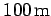above the ground. Suppose, further, that he dropped the first weight straight down, whereas he threw the second weight horizontally with a velocity of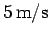. Which weight struck the ground first? How long, after it was thrown, did it take to do this? Finally, what horizontal distance was traveled by the second weight before it hit the ground? Neglect the effect of air resistance.

Answer: Since both weights start off traveling with the same initial velocities in the vertical direction (i.e., zero), and both accelerate vertically downwards at the same rate, it follows that both weights strike the ground simultaneously. The time of flight of each weight is simply the time taken to fall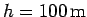, starting from rest, under the influence of gravity. From Eq. (27), this time is given by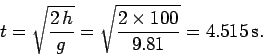The horizontal distancetraveled by the second weight is simply the distance traveled by a body moving at a constant velocity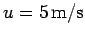(recall that gravitational acceleration does not affect horizontal motion) during the time taken by the weight to drop 100m. Thus,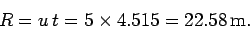Next: Worked example 3.3: Cannon Up: Motion in 3 dimensions Previous: Worked example 3.1: Broken
Richard Fitzpatrick 2006-02-02